# Sample Data: UCI Letter

Letter recognition dataset

This dataset originates from a letter recognition task, where images of the 26 capital letters in the English alphabet should be correctly classified given 16 numerical features of the image.

The example letters were obtained by generating letters using 20 different fonts, which were then randomly distorted to produce 20,000 unique images. Sixteen features (such as pixel counts and correlations) were then extracted from each image.

This dataset comes with predefined test and training sets. The test set consists of the first 16,000 examples with the rest used for training.

## Examples

### Basic Examples

Retrieve the resource:

 In:=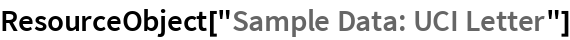Out=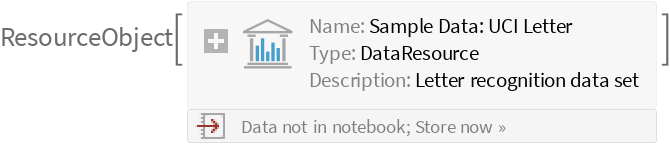Retrieve the default content:

 In:=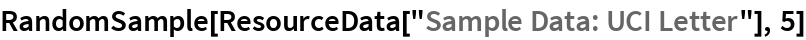Out=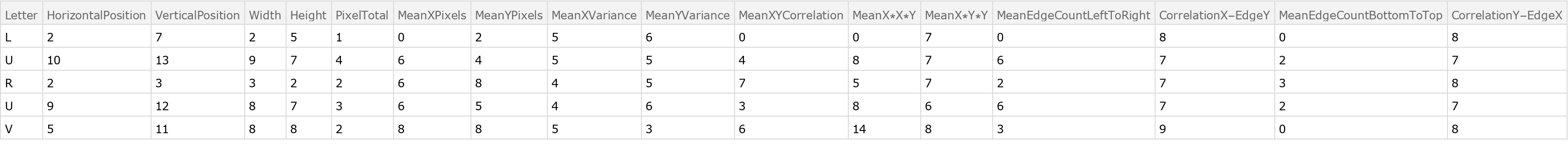### Analysis

Train a classifier:

 In:=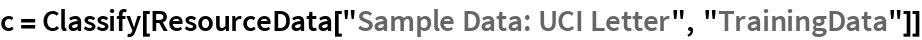Out=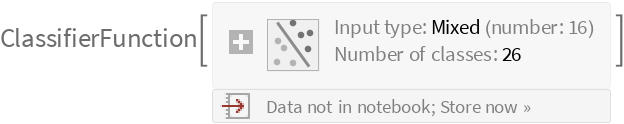Obtain general information about the classifier:

 In:=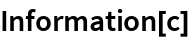Out=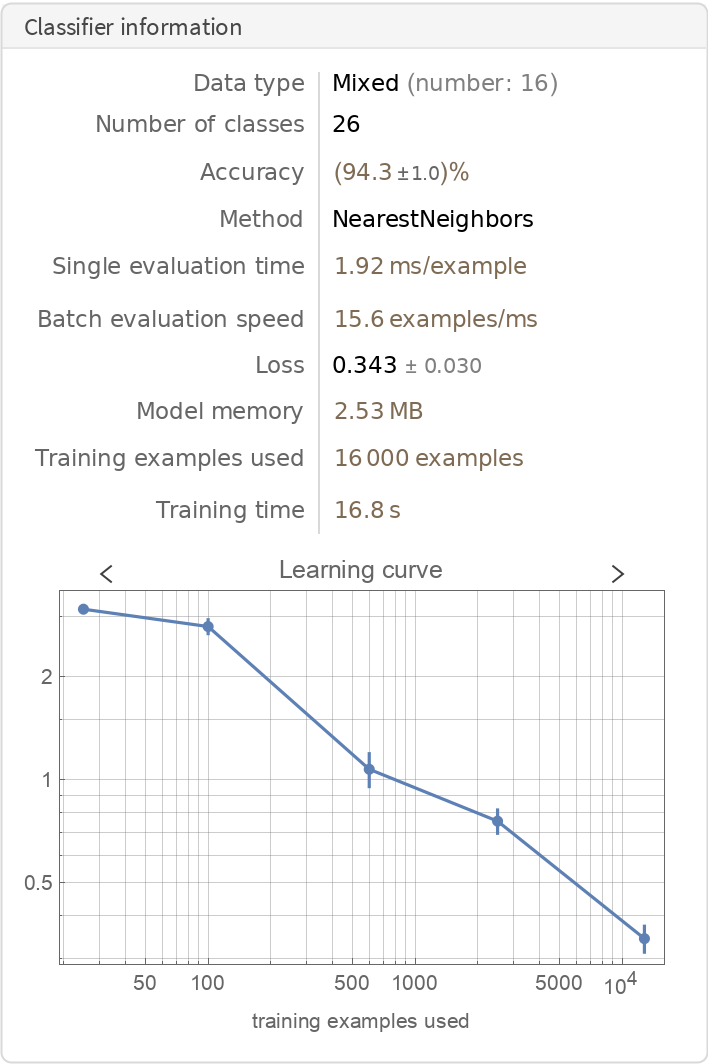Visualize the accuracy of the classifier:

 In:=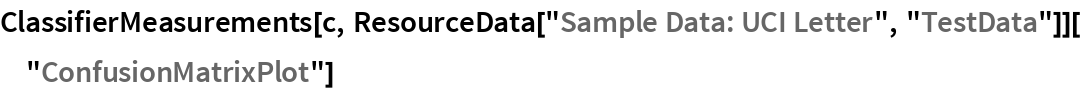Out=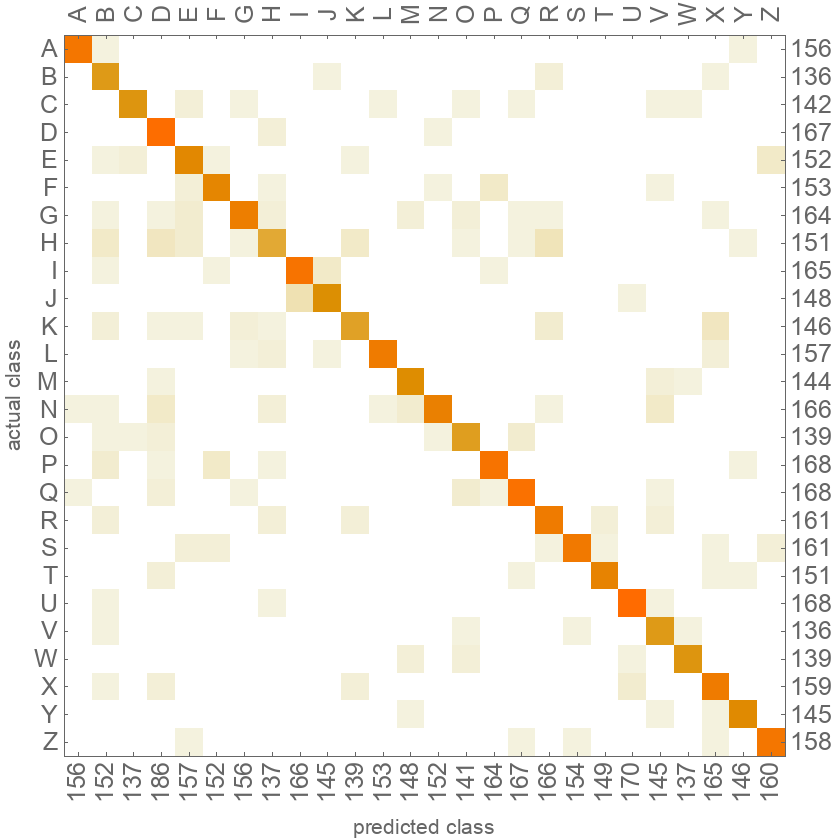Wolfram Research, "Sample Data: UCI Letter" from the Wolfram Data Repository (2019)